A management procedure that incrementally adjusts the TAC to reach a target mean length in catches.

Ltarget1(x, Data, reps = 100, plot = FALSE, yrsmth = 5, xx = 0, xL = 1.05)

Ltarget2(x, Data, reps = 100, plot = FALSE, yrsmth = 5, xx = 0, xL = 1.1)

Ltarget3(x, Data, reps = 100, plot = FALSE, yrsmth = 5, xx = 0, xL = 1.15)

Ltarget4(x, Data, reps = 100, plot = FALSE, yrsmth = 5, xx = 0.2, xL = 1.15)

L95target(x, Data, reps = 100, plot = FALSE, yrsmth = 5, xx = 0, xL = 1.05)

## Arguments

x

A position in the data object

Data

A data object

reps

The number of stochastic samples of the MP recommendation(s)

plot

Logical. Show the plot?

yrsmth

Years over which to calculate mean length.

xx

Parameter controlling the fraction of mean catch to start using in first year

xL

Parameter controlling the magnitude of the target mean length of catches relative to average length in catches.

## Value

An object of class Rec-class with the TAC slot populated with a numeric vector of length reps

## Details

Four target length MPs proposed by Geromont and Butterworth 2014. Tested by Carruthers et al. 2015.

The TAC is calculated as:

If $$L_\textrm{recent} \geq L_0$$: $$\textrm{TAC} = 0.5 \textrm{TAC}^* \left[1+\left(\frac{L_\textrm{recent}-L_0}{L_\textrm{target}-L_0}\right)\right]$$

else: $$\textrm{TAC} = 0.5 \textrm{TAC}^* \left[\frac{L_\textrm{recent}}{L_0}^2\right]$$

where $$\textrm{TAC}^*$$ is (1 - xx) mean catches from the last yrsmth historical years (pre-projection), $$L_\textrm{recent}$$ is mean length in last yrmsth years, $$L_0$$ is (except for L95target) 0.9 average catch in the last 2 x yrsmth historical (pre-projection years) ($$L_\textrm{ave}$$), and $$L_\textrm{target}$$ is (except for L95target) xL $$L_\textrm{ave}$$.

## Functions

• Ltarget1: The least biologically precautionary TAC-based MP.

• Ltarget2: Increasingly biologically precautionary (xL = 1.1).

• Ltarget3: Increasingly biologically precautionary (xL = 1.1).

• Ltarget4: The most biologically precautionary TAC-based MP (xL = 1.1, xx=0.2).

• L95target: Same as Ltarget1 but here the target and limit mean lengths are based on the length at maturity distribution rather than an arbitrary multiplicative of the mean length

## Required Data

See Data-class for information on the Data object

Ltarget1: Cat, LHYear, ML, Year

Ltarget2: Cat, LHYear, ML, Year

Ltarget3: Cat, LHYear, ML, Year

Ltarget4: Cat, LHYear, ML, Year

L95target: Cat, L50, LHYear, ML, Year

## Rendered Equations

See Online Documentation for correctly rendered equations

Other Length target MPs: Lratio_BHI(), LtargetE1()

T. Carruthers

## Examples

Ltarget1(1, Data=MSEtool::SimulatedData, plot=TRUE)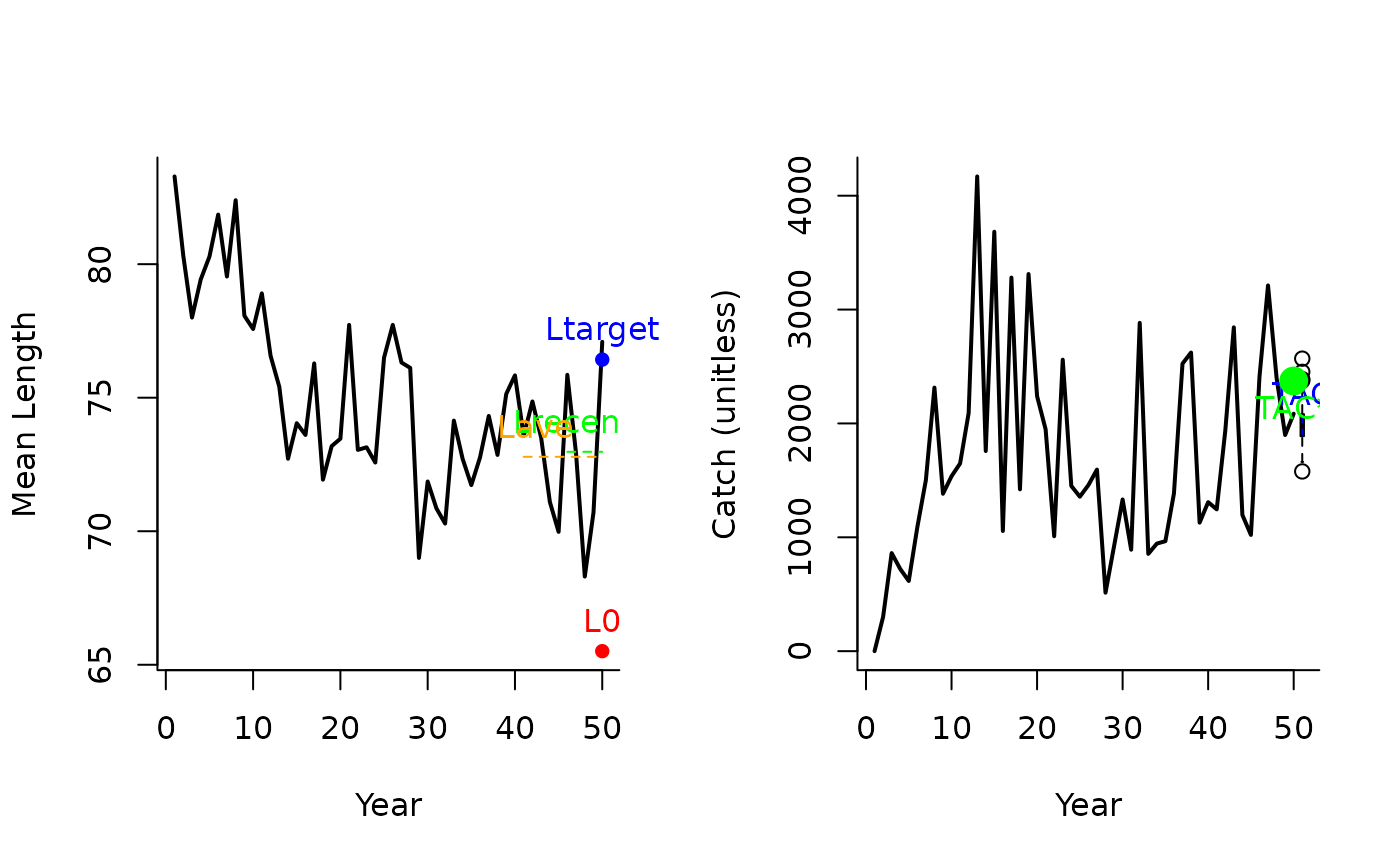#> TAC (median)
#>     2039.194
Ltarget2(1, Data=MSEtool::SimulatedData, plot=TRUE)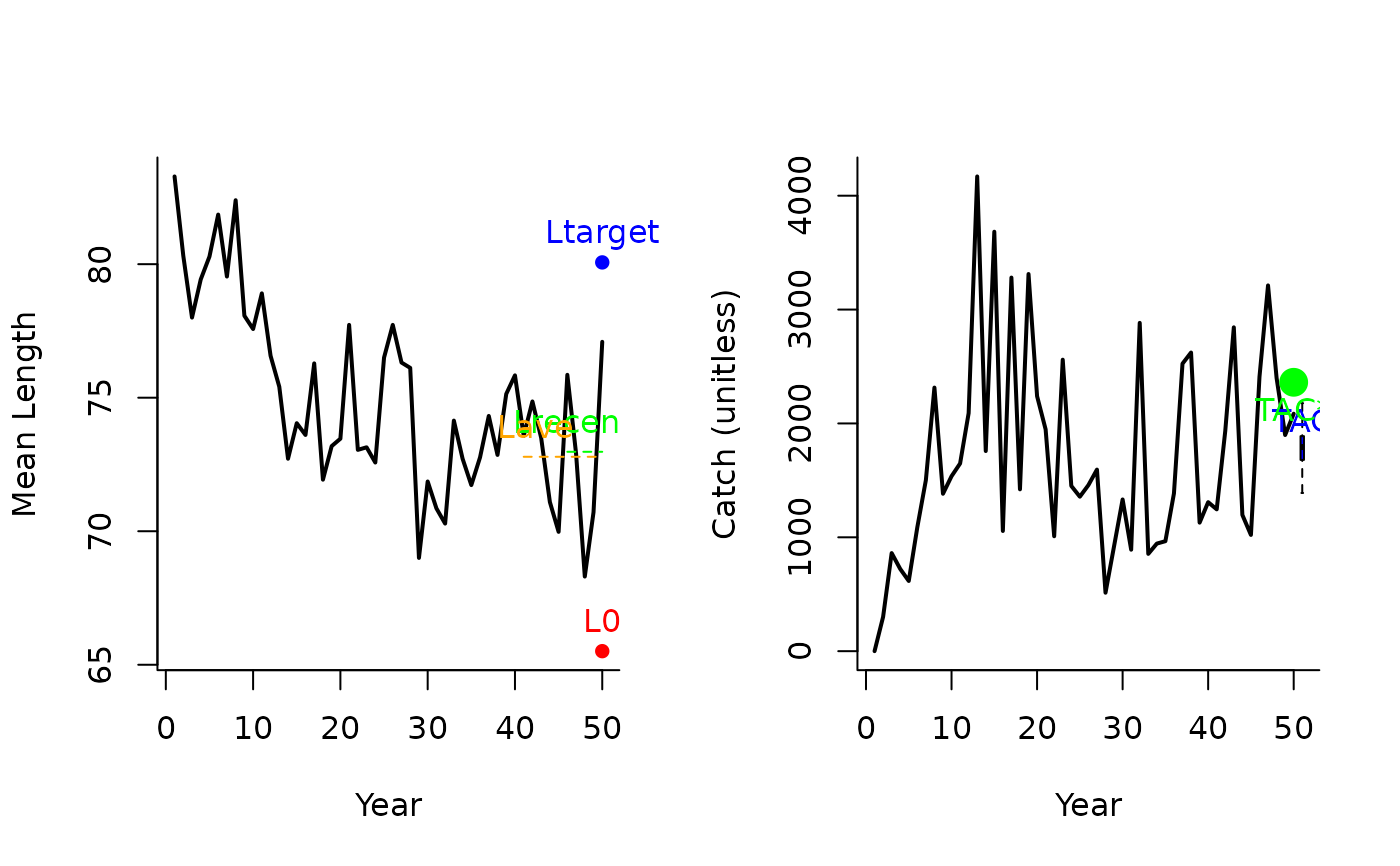#> TAC (median)
#>     1848.201
Ltarget3(1, Data=MSEtool::SimulatedData, plot=TRUE)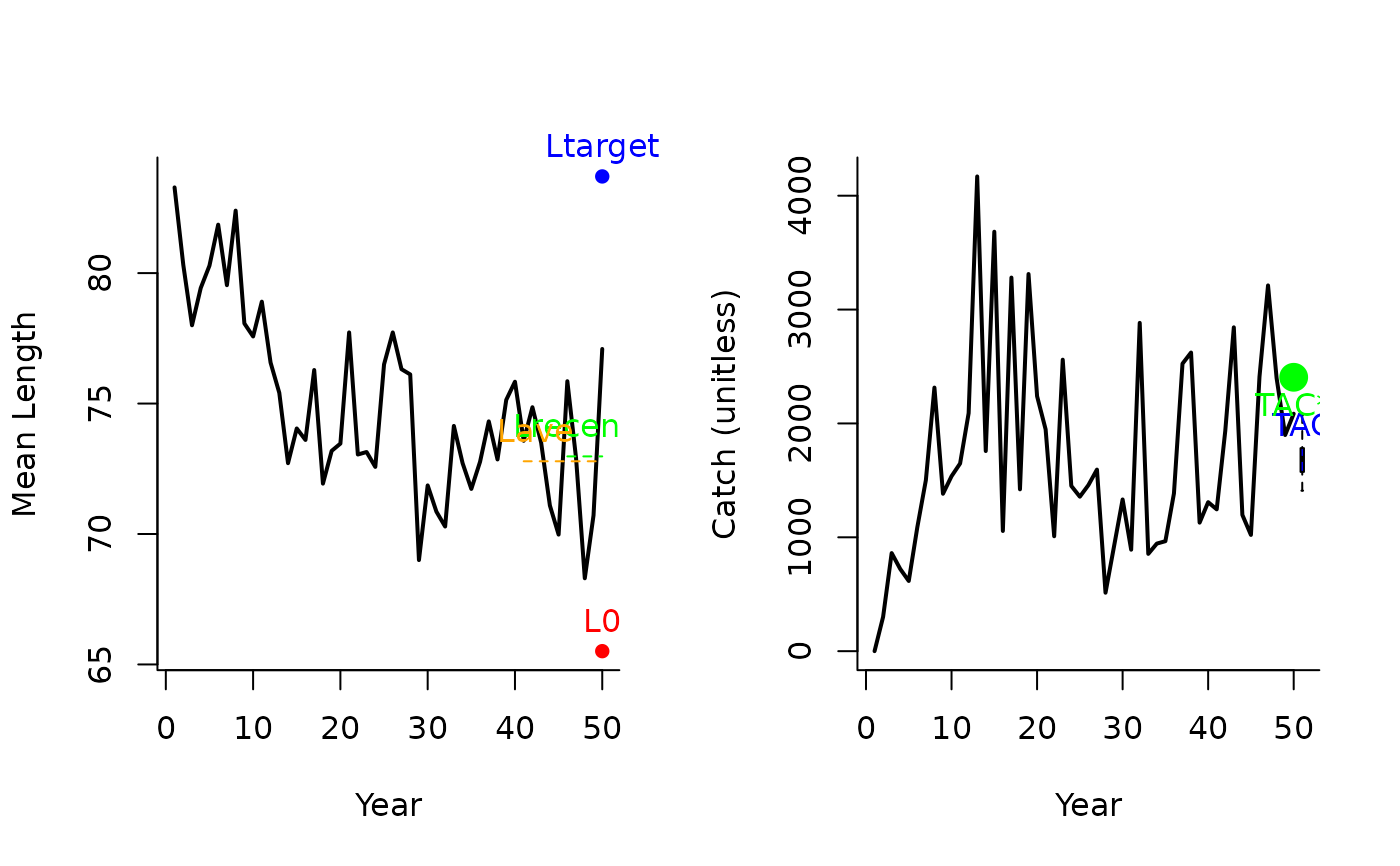#> TAC (median)
#>     1679.592
Ltarget4(1, Data=MSEtool::SimulatedData, plot=TRUE)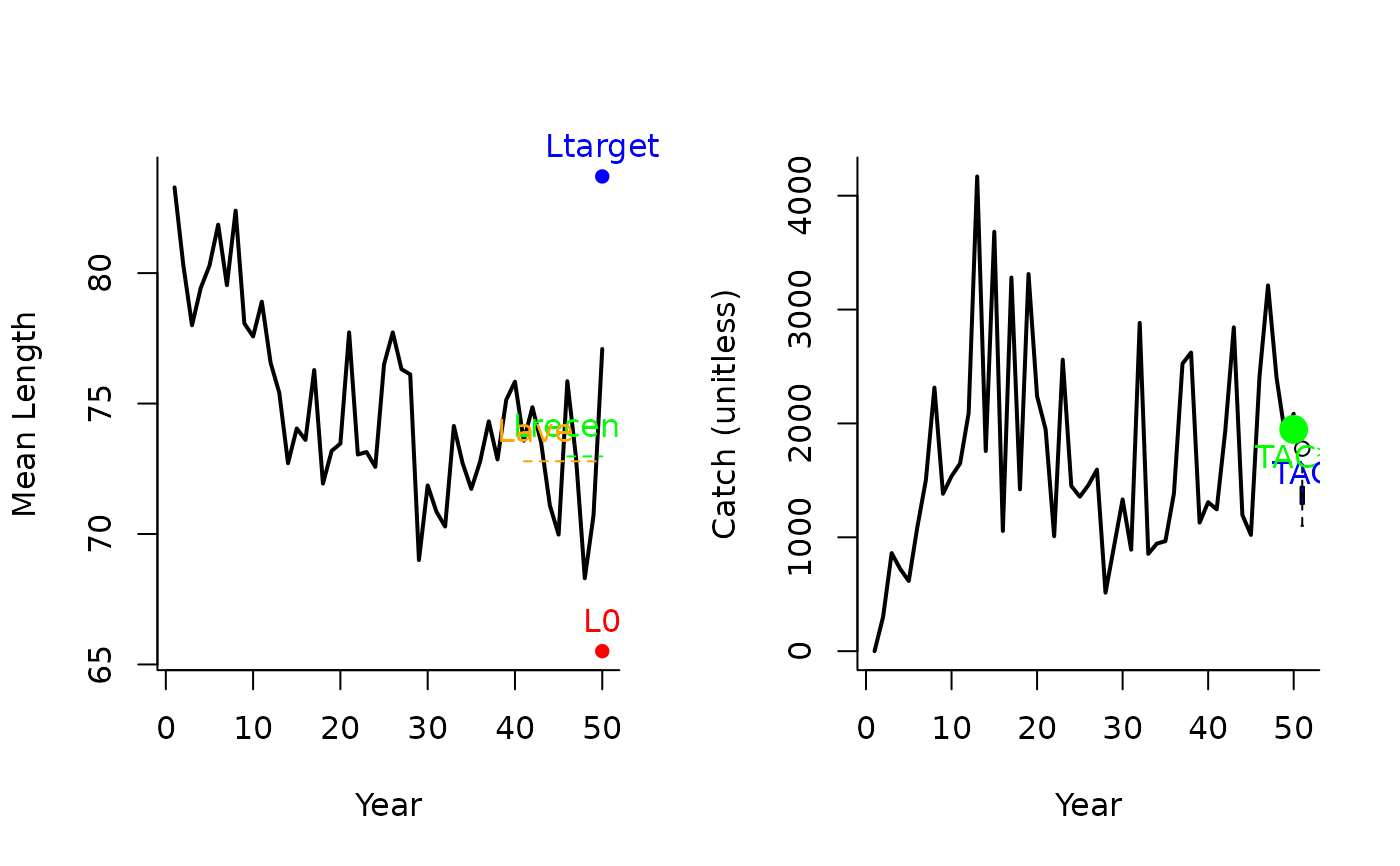#> TAC (median)
#>     1329.029
L95target(1, Data=MSEtool::SimulatedData, plot=TRUE)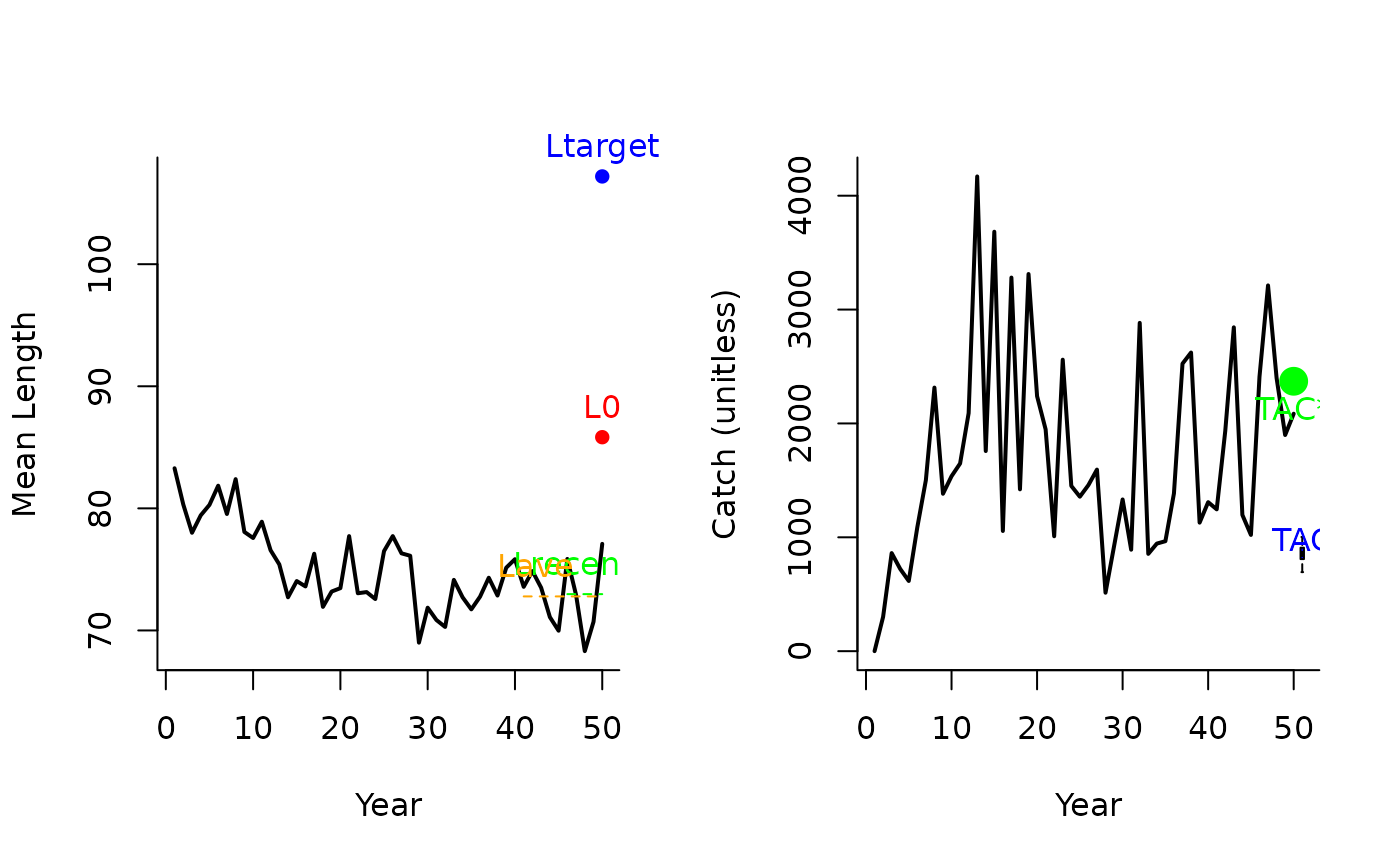#> TAC (median)
#>     862.7857